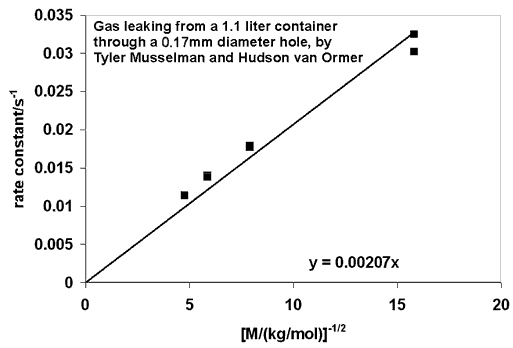# Experiment of The Month

## When Equipartition of Energy Does Not Work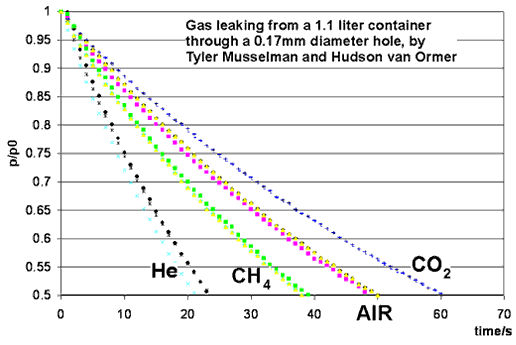This month's experiment is performed by students in the Chemistry Department's physical chemistry course, taught by Dr. Iannone. Students were Tyler Musselman, Hudson van Ormer, Ian Rowe, Kelly Rhoads, Anthony Burkholder and Erik Allman. A small hole is opened in a container of gas, and the pressure of the gas is measured as a function of time. The ideal gas laws give an excellent prediction for the rate at which gas leaks out, provided that the average velocity of gas molecules is correctly calculated.

The figures below show enlarging views of the opening and the trajectory of an escaping gas molecule.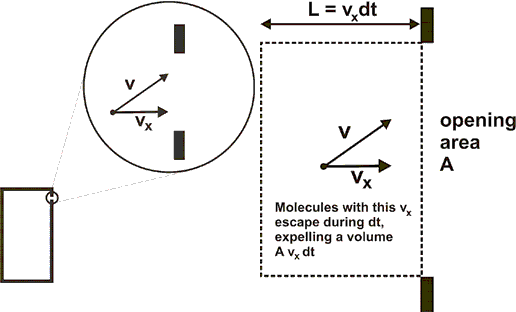Velocity component vx in the figure is the component that carries molecules out of the opening. During time dt,
dN=(r)(Avxdt) move out of the volume. (r is the number of molecules per unit volume.)

The rate at which molecules with this vx leave the volume is dN/dt = rAvx

If that is the average velocity component, we can use it to estimate the average rate at which molecules leave the volume. We can find the average velocity component in terms of in terms of temperature, T, in two interesting ways.

The naive approach is to refer to the equipartition of energy, stating that each degree of freedom of a molecule carries, on average, 1/3 of the total energy of the molecule. That is, m <vx2>=kT

where m is the mass of the molecule and k is Boltzmann's constant, and the <> brackets indicate the average value of vx2. Naively, we can set
vx=(kT/m)(1/2).

However, for this problem we need the average of the velocity component, which is different from the average of the square of the velocity component. The correct approach is to use the Boltzmann velocity distribution. This distribution correctly leads to equipartition of energy, and it also leads, separately, to the correct average velocity component in the positive x direction.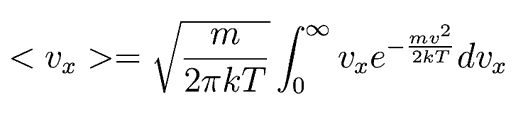Thanks to Michael Young for pointing out the need for the normalization factor under the square root.

Following Hyperphysics pages for these kinds of integrals, we can find that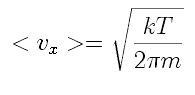In the equation representing outflow of the gas, N is hard to measure, but the pressure in the constant volume, constant temperature serves as an indicator for N:

This integrates to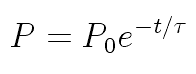where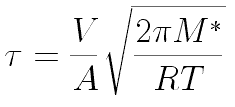and we have changed from the mass of a molecule, m, to its molecular "weight," M*.

Dr. Iannone's students Ian Rowe and Kelly Rhoads measured 90 seconds for t when the gas was carbon dioxide. They predicted, for their 0.39mm diameter hole, a characteristic time, t, of 88.5 seconds.

When the naive value for vx is used for carbon dioxide, the predicted t is only 35 seconds. The Boltzmann distribution correctly gives both equipartition of energy and the average velocity component. Using equipartition of energy to estimate the average velocity component is incorrect because the energy uses the average of the square of the velocity.

The graph below shows the rate constant (k = 1/t) versus 1/(square root of molecular weight) for the data graphed at the top of the page. The results indicate that t does indeed depend on the square root of the molecular weight, as predicted. They also hint at a discrepancy for helium, the pair of points farthest right on the graph. It would be interesting if the long mean free path of helium molecules were being reflected in the data.